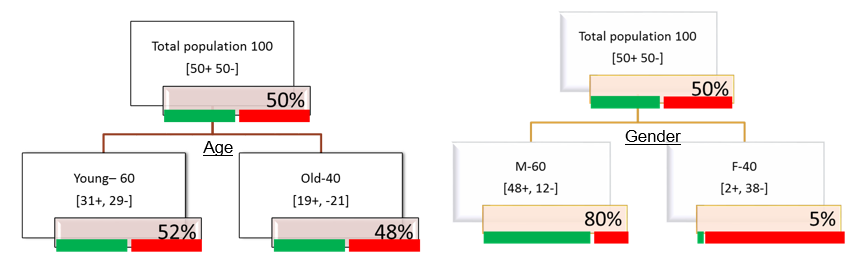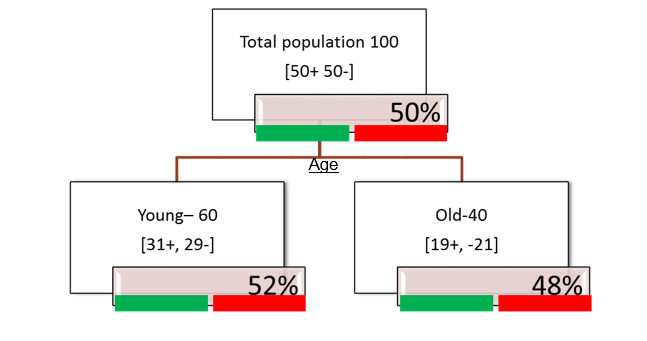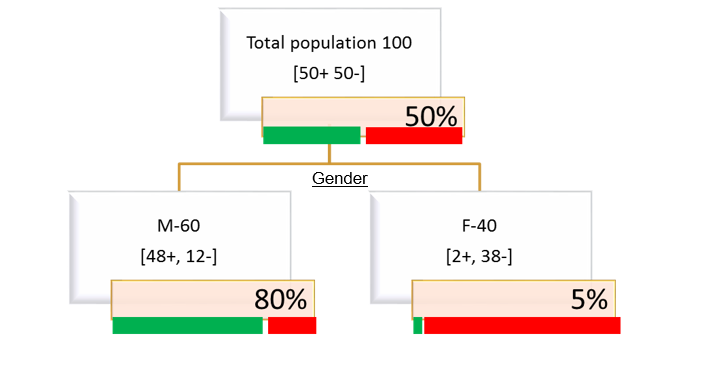• No products in the cart.

# 204.3.3 How Decision tree Splits works?

##### Split criterion of decision tree algorithms.

Link to the previous post : https://statinfer.com/204-3-2-the-decision-tree-approach/

Decision Tree follows the Algorithm ID3 (Iterative Dichotomiser 3). This algorithm iteratively splits data into segments which have a decrease in Entropy and increase in Information Gain with each split.

The final goal is to achieve homogeneity in final nodes.

Two matrices of Decision Tree Algorithms are:

1. Entropy :  is the uncertainty in the data point which we want to decrease with each split.
2. Information Gain : is the decrease in Entropy after each split, which we want to increase with each split.

We shall cover Entropy in this post and see how it can be calculated.

### Impurity (Diversity) Measures

• We are looking for a impurity or diversity measure that will give high score for this Age variable(high impurity while segmenting), Low score for Gender variable(Low impurity while segmenting).• Entropy: Characterizes the impurity/diversity of segment.
• Measure of uncertainty/Impurity.
• Entropy measures the information amount in a message.
• S is a segment of training examples, p+ is the proportion of positive examples, p- is the proportion of negative examples.
• Entropy(S)=−(p+)(log2(p+))−(p−)(log2(p−))
• Where p+ is the probability of positive class and pis the probability of negative class.
• Entropy is highest when the split has p of 0.5.
• Entropy is least when the split is pure .ie p of 1.

### Entropy is highest when the split has p of 0.5

• Entropy(S)=−(p+)(log2(p+))−(p−)(log2(p−))
• Entropy is highest when the split has p of 0.5
• 50-50 class ratio in a segment is really impure, hence entropy is high
• Entropy(S)=−(p+)(log2(p+))−(p−)(log2(p−))
• Entropy(S) = 0.5log2(0.5)0.5log2(0.5)
• Entropy(S) = 1### Entropy is least when the split is pure .ie p of 1

• Entropy(S)=−(p+)(log2(p+))−(p−)(log2(p−))
• Entropy is least when the split is pure ie p of 1
• 100-0 class ratio in a segment is really pure, hence entropy is low
• Entropy(S)=−(p+)(log2(p+))−(p−)(log2(p−))
• Entropy(S) = 1log2(1)0log2(0)
• Entropy(S) = 0### The less the entropy, the better the split

• The less the entropy, the better the split.
• Entropy is formulated in such a way that, its value will be high for impure segments.

In next post we will see how to calculate the entropy for each split.

The next post is about how to calculate entropy for decision tree split.

Link to the next post : https://statinfer.com/204-3-4-how-to-calculate-entropy-for-decision-tree-split/

24th January 2018